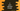# C program to print an alphabet triangle with user-input height## C program to print alphabet triangle:

Let’s learn how to print an alphabet triangle or a triangle with alphabet in C. It will take the height of the triangle as input from the user and print the pattern with alphabet. Before we start with the program, let’s learn how the algorithm works.

### Algorithm to print an alphabet pattern triangle:

To understand the program, we have to learn the algorithm first. For example, a pattern of height 5 looks as below:

``````    A
A B
A B C
A B C D
A B C D E``````

We are using characters and blank spaces to print this pattern. Let me replace all blank spaces with *:

``````****A
***A B
**A B C
*A B C D
A B C D E``````

As you can see here, it prints:

• 4 blank spaces and 1 character for the first line.
• 3 blank spaces and 2 characters for the second line.
• 2 blank spaces and 3 characters for the third line etc.

We can use 3 loops to print this pattern: 1 outer loop and 2 inner loops. The outer loop will point to each row of the pattern, the first inner loop will print the blank spaces and the second inner loop will print the characters of the pattern.

Let’s say, the height of the triangle is stored in the variable height.

• The first loop will run from i = 1 to i = height.
• The first inner loop will run from j = 1 to j = height - i. This loop will print the starting blank spaces of each row.
• The second inner loop will run from k = 1 to k = i. This loop will print the characters.
• At the end of each iteration of the outer loop, we will have to print one new line to move to the next line.

### Example 1: C program to print a triangle with alphabet:

Let’s use the above algorithm to write down the program in C:

``````#include <stdio.h>

int main()
{
int height, c = 64;

printf("Enter the height of the triangle: ");
scanf("%d", &height);

for (int i = 1; i <= height; i++)
{
for (int j = 1; j <= height - i; j++)
{
printf(" ");
}
for (int k = 1; k <= i; k++)
{
printf("%c ", c + k);
}
printf("\n");
}

return 0;
}``````

Here,

• height and c are two integer variables to hold the height of the triangle and we can add the value of c with any other integer value to print the alphabet character. For example, 65 is the ASCII value of A, 66 is the ASCII value of B, etc.
• The outer for loop runs from i = 1 to i = height. On each iteration of this loop, it runs two more loops to print the blank spaces and characters.
• The first inner loop runs from j = 1 to j = height. It is used to print the blank spaces.
• The second inner loop runs from k = 1 to k = i. It prints the character with the ASCII value of c + k.
• At the end of each iteration of the outer loop, it prints a newline character.

If you run the program, it will print output as below:

``````Enter the height of the triangle: 6
A
A B
A B C
A B C D
A B C D E
A B C D E F ``````

### Example 2: C program to print a triangle with alphabet using while loops:

Let’s write the above program with while loops. We will use the same algorithm and variables but all for loops will be replaced by while loops:

``````#include <stdio.h>

int main()
{
int height, c = 64, i = 1, j, k;

printf("Enter the height of the triangle: ");
scanf("%d", &height);

while (i <= height)
{
j = 1;
k = 1;

while (j <= height - i)
{
printf(" ");
j++;
}
while (k <= i)
{
printf("%c ", c + k);
k++;
}
printf("\n");
i++;
}

return 0;
}``````

It will print similar output.

``````Enter the height of the triangle: 6
A
A B
A B C
A B C D
A B C D E
A B C D E F``````